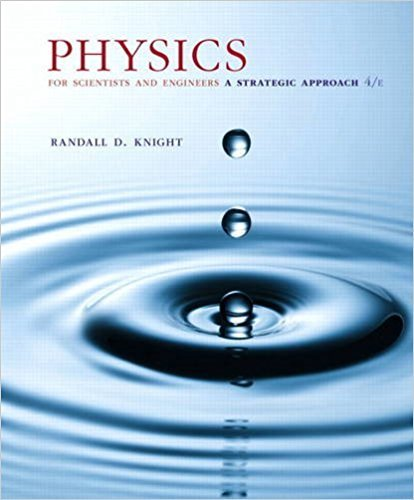×
×

# Use the data from Figure 40.24 to calculate the firstISBN: 9780134081496 191

## Solution for problem 40.54 Chapter 40

Physics for Scientists and Engineers: A Strategic Approach, Standard Edition (Chs 1-36) | 4th Edition

• Textbook Solutions
• 2901 Step-by-step solutions solved by professors and subject experts
• Get 24/7 help from StudySoup virtual teaching assistantsPhysics for Scientists and Engineers: A Strategic Approach, Standard Edition (Chs 1-36) | 4th Edition

4 5 0 263 Reviews
17
3
Problem 40.54

Use the data from Figure 40.24 to calculate the first three vibrational energy levels of a C= O carbon-oxygen double bond

Step-by-Step Solution:
Step 1 of 3

Chapter 31: Atomic Physics  Line spectrum of hydrogen: a series of individual lines caused by light being emitted by a low­pressure gas when subjected to a large electric field.  Wavelengths in the visible spectrum of hydrogen are given by the following equation: 1 =R 1 − 1 λ (2) n2 where n = 3, 4, 5,… and where R is the Rydberg constant...

Step 2 of 3

Step 3 of 3

##### ISBN: 9780134081496

Physics for Scientists and Engineers: A Strategic Approach, Standard Edition (Chs 1-36) was written by and is associated to the ISBN: 9780134081496. This textbook survival guide was created for the textbook: Physics for Scientists and Engineers: A Strategic Approach, Standard Edition (Chs 1-36), edition: 4. The answer to “Use the data from Figure 40.24 to calculate the first three vibrational energy levels of a C= O carbon-oxygen double bond” is broken down into a number of easy to follow steps, and 21 words. This full solution covers the following key subjects: . This expansive textbook survival guide covers 42 chapters, and 4463 solutions. Since the solution to 40.54 from 40 chapter was answered, more than 225 students have viewed the full step-by-step answer. The full step-by-step solution to problem: 40.54 from chapter: 40 was answered by , our top Physics solution expert on 12/28/17, 08:06PM.

Unlock Textbook Solution